Batch Normalization深入理解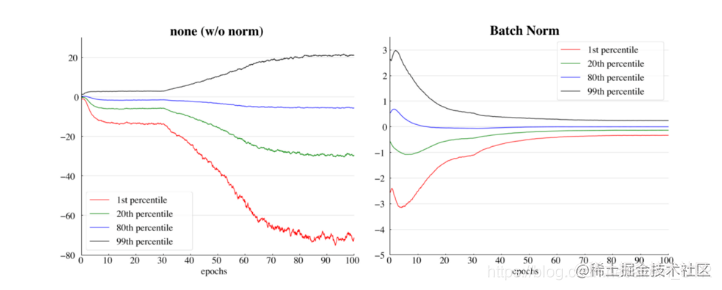(左)没有任何归一化，(右)应用了batch normalization

2. BN工作原理是什么？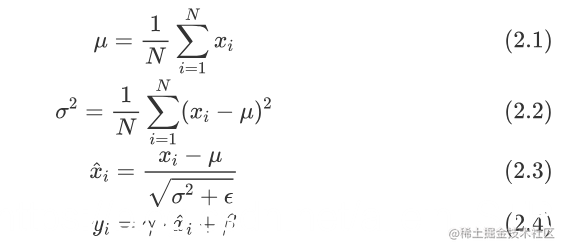BN层通常添加在隐藏层的激活函数之前，线性变换之后。如果我们把(2.4)和之后的激活函数放在一起看，可以将他们视为一层完整的神经网络（线性+激活）。（注意BN的线性变换和一般隐藏层的线性变换仍有区别，前者是element-wise的，后者是矩阵乘法。）

3. BN实现方法是什么？

import numpy as np

def batchnorm_forward(x, gamma, beta, bn_param):
N, D = x.shape
eps = bn_param.get('eps', 1e-5)
momentum = bn_param.get('momentum', 0.9)
running_mean = bn_param.get('running_mean', np.zeros(D, dtype=x.dtype))
running_var = bn_param.get('running_var', np.zeros(D, dtype=x.dtype))

# BN forward pass
sample_mean = x.mean(axis=0)
sample_var = x.var(axis=0)
x_ = (x - sample_mean) / np.sqrt(sample_var + eps)
out = gamma * x_ + beta

# update moving average
running_mean = momentum * running_mean + (1-momentum) * sample_mean
running_var = momentum * running_var + (1-momentum) * sample_var
bn_param['running_mean'] = running_mean
bn_param['running_var'] = running_var

# storage variables for backward pass
cache = (x_, gamma, x - sample_mean, sample_var + eps)

return out, cache

def batchnorm_backward(dout, cache):
# extract variables
N, D = dout.shape
x_, gamma, x_minus_mean, var_plus_eps = cache

dgamma = np.sum(x_ * dout, axis=0)
dbeta = np.sum(dout, axis=0)

dx_ = np.matmul(np.ones((N,1)), gamma.reshape((1, -1))) * dout
dx = N * dx_ - np.sum(dx_, axis=0) - x_ * np.sum(dx_ * x_, axis=0)
dx *= (1.0/N) / np.sqrt(var_plus_eps)

return dx, dgamma, dbeta

• 更快的收敛。
• 降低初始权重的重要性。
• 鲁棒的超参数。
• 需要较少的数据进行泛化。

5. BN缺点是什么？

• 在使用小batch size的时候不稳定： batch normalization必须计算平均值和方差，以便在batch中对之前的输出进行归一化。如果batch大小比较大的话，这种统计估计是比较准确的，而随着batch大小的减少，估计的准确性持续减小。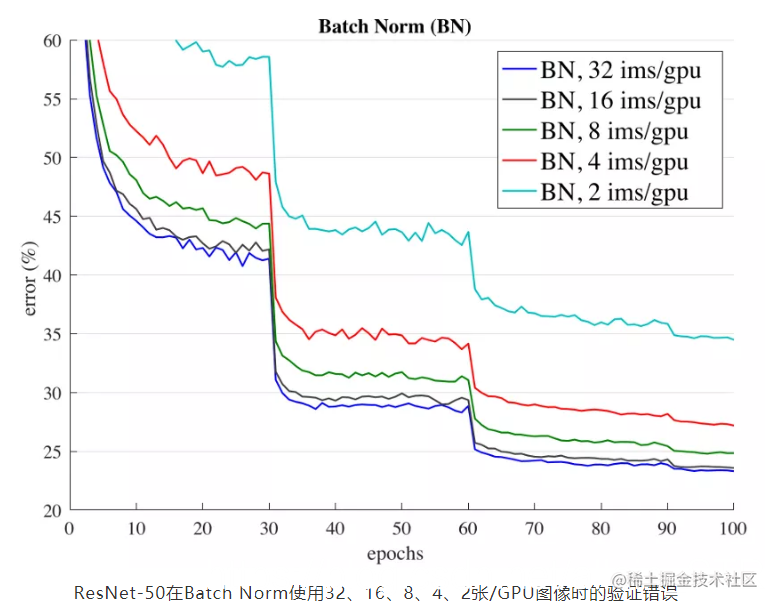• 导致训练时间的增加：NVIDIA和卡耐基梅隆大学进行的实验结果表明，“尽管Batch Normalization不是计算密集型，而且收敛所需的总迭代次数也减少了。”但是每个迭代的时间显著增加了，而且还随着batch大小的增加而进一步增加。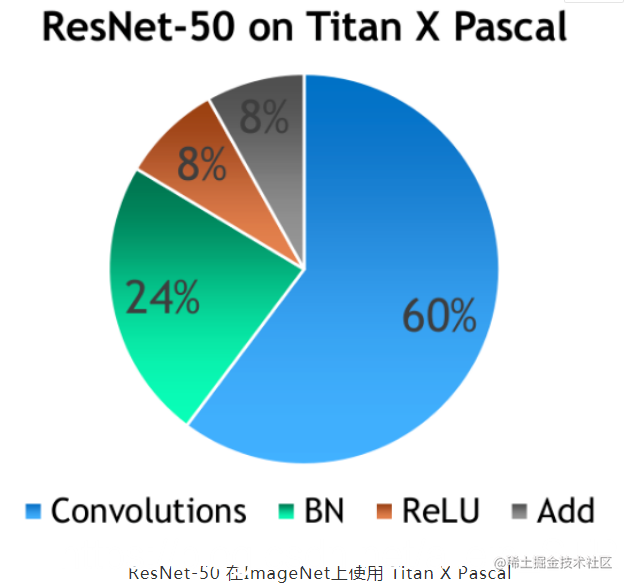batch normalization消耗了总训练时间的1/4。原因是batch normalization需要通过输入数据进行两次迭代，一次用于计算batch统计信息，另一次用于归一化输出。

• 训练和推理时不一样的结果：例如，在真实世界中做“物体检测”。在训练一个物体检测器时，我们通常使用大batch(YOLOv4和Faster-RCNN都是在默认batch大小= 64的情况下训练的)。但在投入生产后，这些模型的工作并不像训练时那么好。这是因为它们接受的是大batch的训练，而在实时情况下，它们的batch大小等于1，因为它必须一帧帧处理。考虑到这个限制，一些实现倾向于基于训练集上使用预先计算的平均值和方差。另一种可能是基于你的测试集分布计算平均值和方差值。
• 对于在线学习不好：在线学习是一种学习技术，在这种技术中，系统通过依次向其提供数据实例来逐步接受训练，可以是单独的，也可以是通过称为mini-batch的小组进行。每个学习步骤都是快速和便宜的，所以系统可以在新的数据到达时实时学习。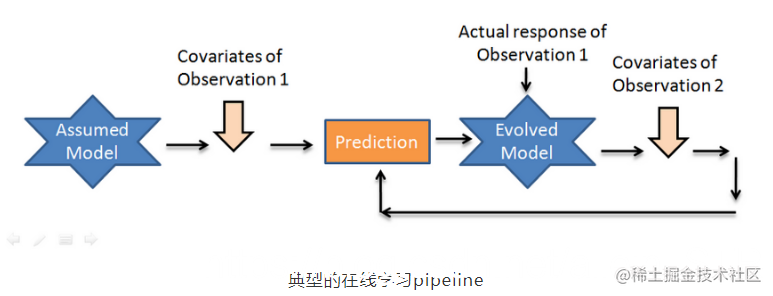• 对于循环神经网络不好：

虽然batch normalization可以显著提高卷积神经网络的训练和泛化速度，但它们很难应用于递归结构。batch normalization可以应用于RNN堆栈之间，其中归一化是“垂直”应用的，即每个RNN的输出。但是它不能“水平地”应用，例如在时间步之间，因为它会因为重复的重新缩放而产生爆炸性的梯度而伤害到训练。

[^注]: 一些研究实验表明，batch normalization使得神经网络容易出现对抗漏洞，但我们没有放入这一点，因为缺乏研究和证据。

6. 可替换的方法：

• Layer Normalization
• Instance Normalization
• Group Normalization (+ weight standardization)
• Synchronous Batch Normalization

7.参考资料

1. Batch Normalization: Accelerating Deep Network Training by Reducing Internal Covariate Shift
2. Deriving the Gradient for the Backward Pass of Batch Normalization
3. CS231n Convolutional Neural Networks for Visual Recognition
4. towardsdatascience.com/curse-of-ba…